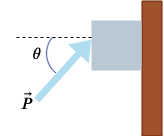# A block of mass 2.25 kg is pushed up against a wall by a force \vec P that makes an angle of...

## Question:

A block of mass 2.25 kg is pushed up against a wall by a force {eq}\vec P {/eq} that makes an angle of {eq}\theta = 50.0^\circ {/eq} angle with the horizontal as shown below. The coefficient of static friction between the block and the wall is 0.228.(a) Determine the possible values for the magnitude of {eq}\vec P {/eq} that allow the block to remain stationary. (If there is no maximum, enter NONE in the answer blank.)

{eq}\left | \vec P_{max} \right | = {/eq} _____ N

{eq}\left | \vec P_{min} \right | = {/eq} _____ N

(b) What happens if {eq}\left | \vec P \right | {/eq} has a larger value than {eq}\left | \vec P_{max}\right |? {/eq}

{eq}\bigcirc {/eq} The block slides up the wall.

{eq}\bigcirc {/eq} The block does not slide along the wall.

{eq}\bigcirc {/eq} The block slides down the wall.

What happens if {eq}\left | \vec P \right | {/eq} has a smaller value than {eq}\left | \vec P_{min}\right |? {/eq}

{eq}\bigcirc {/eq} The block slides up the wall.

{eq}\bigcirc {/eq} The block does not slide along the wall.

{eq}\bigcirc {/eq} The block slides down the wall.

(c) Repeat parts (a) and (b) assuming the force makes an angle of {eq}\theta = 15^\circ {/eq} with the horizontal.

Determine the possible values for the magnitude of {eq}\vec P {/eq} that allow the block to remain stationary. (If there is no maximum, enter NONE in the answer blank.)

{eq}\left | \vec P_{max} \right | = {/eq} _____ N

{eq}\left | \vec P_{min} \right | = {/eq} _____ N

What happens if {eq}\left | \vec P \right | {/eq} has a larger value than {eq}\left | \vec P_{max}\right |? {/eq}

{eq}\bigcirc {/eq} The block slides up the wall.

{eq}\bigcirc {/eq} The block does not slide along the wall.

{eq}\bigcirc {/eq} The block slides down the wall.

What happens if {eq}\left |\vec P \right | {/eq} has a smaller value than {eq}\left | \vec P_{min}\right |? {/eq}

{eq}\bigcirc {/eq} The block slides up the wall.

{eq}\bigcirc {/eq} The block does not slide along the wall.

{eq}\bigcirc {/eq} The block slides down the wall.

## Friction:

When a body is moved along a rough surface having the coefficient of friction {eq}\mu {/eq} then the frictional force acts on the body is {eq}f_r = \mu N {/eq}.

• where N is the normal reaction acting on the body

The frictional force acts in the direction opposite to the motion of the body.

Become a Study.com member to unlock this answer! Create your account

PART 1

A block of mass m = 2.25 kg is pushed up against a wall by a force that makes an angle of {eq}\theta {/eq} = 50.0{eq}^\circ {/eq} below the...Sliding Friction: Definition, Formula & Examples

from

Chapter 11 / Lesson 23
38K

Sliding friction is different than static friction for most material combinations. Find out how it differs, when to apply this concept, and how to calculate the forces involved in this lesson.